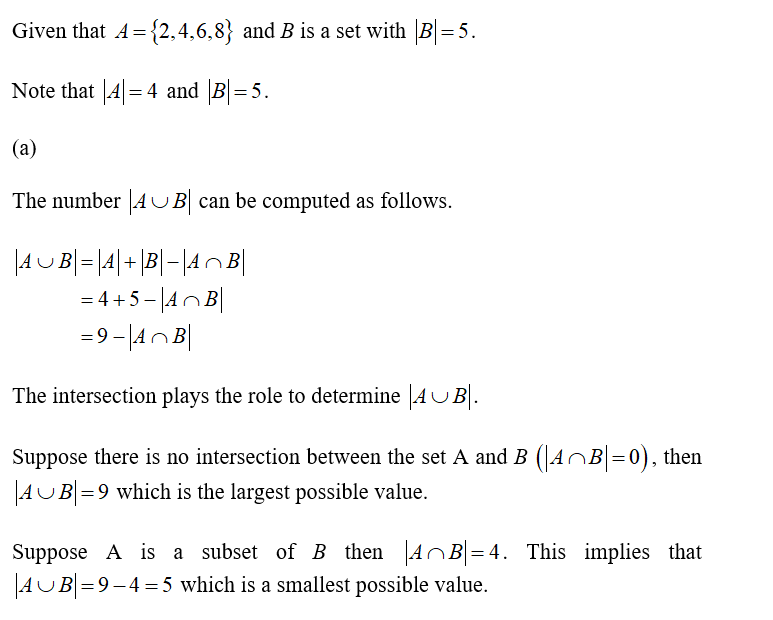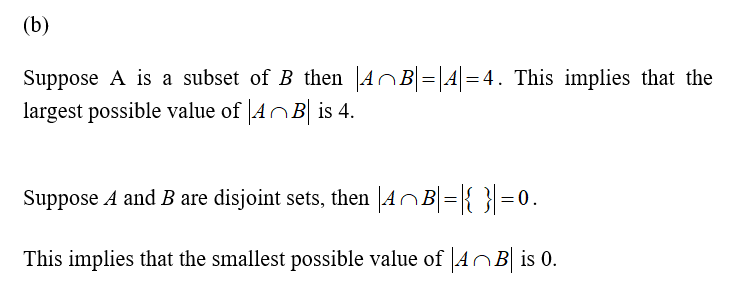# 2. Let A = {2,4,6,8}. Suppose B is a set with |B| = 5.(a) What are the smallest and largest possible values of |A∪B|? Explain.(b)  What are the smallest and largest possible values of |A∩B|? Explain.(c)  What are the smallest and largest possible values of |A×B|? Explain.

Question
2 views

2. Let A = {2,4,6,8}. Suppose B is a set with |B| = 5.
(a) What are the smallest and largest possible values of |A∪B|? Explain.
(b)  What are the smallest and largest possible values of |A∩B|? Explain.
(c)  What are the smallest and largest possible values of |A×B|? Explain.

check_circle

Step 1Step 2...

### Want to see the full answer?

See Solution

#### Want to see this answer and more?

Solutions are written by subject experts who are available 24/7. Questions are typically answered within 1 hour.*

See Solution
*Response times may vary by subject and question.
Tagged in